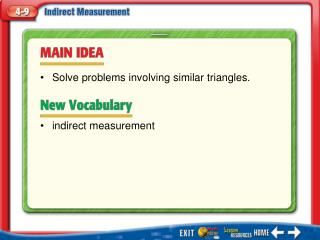# Main Idea/Vocabulary - PowerPoint PPT PresentationDownload PresentationMain Idea/Vocabulary

Main Idea/VocabularyDownload Presentation## Main Idea/Vocabulary

- - - - - - - - - - - - - - - - - - - - - - - - - - - E N D - - - - - - - - - - - - - - - - - - - - - - - - - - -
##### Presentation Transcript

1. Solve problems involving similar triangles. • indirect measurement Main Idea/Vocabulary

2. Use Shadow Reckoning TREES A tree in front of Marcel’s house has a shadow 12 feet long. Marcel’s shadow is 3 feet long. If Marcel is 5.5 feet tall, how tall is the tree? Example 1

3. tree’s shadow tree’s height Marcel’s shadow Marcel’s height Use Shadow Reckoning 12 ● 5.5 = 3 ● h Find the cross products. Multiply. Divide each side by 3. Simplify. Answer: The tree is 22 feet tall. Example 1

4. A B C D Jayson casts a shadow that is 10 feet long. At the same time, a flagpole casts a shadow that is 40 feet long. If the flagpole is 20 feet tall, how tall is Jayson? A. 4.5 feet B. 5 feet C. 5.5 feet D. 6 feet Example 1

5. Use Indirect Measurement SURVEYING The two triangles shown in the figure are similar. Find the distance d across the stream. Example 2

6. Use Indirect Measurement Write a proportion. AB= 48, ED=d, BC = 60, and DC = 20 48 ● 20 = d ● 60 Find the cross products. Multiply. Then divide each side by 60. Simplify. Answer: The distance across the stream is 16 meters. Example 2

7. A B C D SURVEYINGThe two triangles shown in the figure are similar. Find the distance d across the stream. A. 6 feet B. 6.5 feet C. 7 feet D. 7.5 feet Example 2

8. A B C D (over Lesson 4-8) Find the coordinates of the image of the parallelogram ABCD after a dilation with a scale factor of 2. Parallelogram ABCD has vertices at A(1, 1), B(3, 3), C(6, 3), D(4, 1). A. A′(2, 2), B′(6, 6), C′(12, 6), D′(8, 2) B. A′(1, 2), B′(5, 6), C′(12, 12), D′(8, 2) C. A′(6, 6), B′(2, 2), C′(12, 6), D′(2, 8) D.A′(2, 2), B′(6, 6), C′(6, 12), D′(8, 2) Five Minute Check 1

9. A B C D A. B. C. D. (over Lesson 4-8) Five Minute Check 2

10. A B C (over Lesson 4-8) Parallelogram ABCD has vertices at A(1, 1), B(3, 3), C(6, 3), D(4, 1). Identify whether the image of parallelogram ABCD after a dilation with a scale factor of 2 is an enlargement or a reduction. A. enlargement B. reduction C. cannot be determined Five Minute Check 3

11. A B C (over Lesson 4-8) A. enlargement B. reduction C. cannot be determined Five Minute Check 4

12. A B C D A. 3 B. 2 C. D. (over Lesson 4-8) Triangle M is similar to triangle N. What scale factor was used to dilate triangle N to M? Five Minute Check 5

13. End of Custom Shows